• 累计撰写 270 篇文章
• 累计创建 6 个标签
• 累计收到 1 条评论

### 目 录CONTENT# LFU缓存详解.md2021-08-22 / 0 评论 / 0 点赞 / 6 阅读 / 5,674 字 / 正在检测是否收录...

# LFU缓存详解

@[toc]

## 一、LFU概念

•  LRU 算法相当于把数据按照时间排序，这个需求借助链表很自然就能实现，你一直从链表头部加入元素的话，越靠近头部的元素就是新的数据，越靠近尾部的元素就是旧的数据，我们进行缓存淘汰的时候只要简单地将尾部的元素淘汰掉就行了。

•  LFU 算法相当于是淘汰访问频次最低的数据如果访问频次最低的数据有多条，需要淘汰最旧的数据。把数据按照访问频次进行排序，而且频次还会不断变化，这可不容易实现。

•  从题面上感觉并不难，不就是排个序嘛，如果这么想就错了：因为要求O(1) 时间复杂度内执行两项操作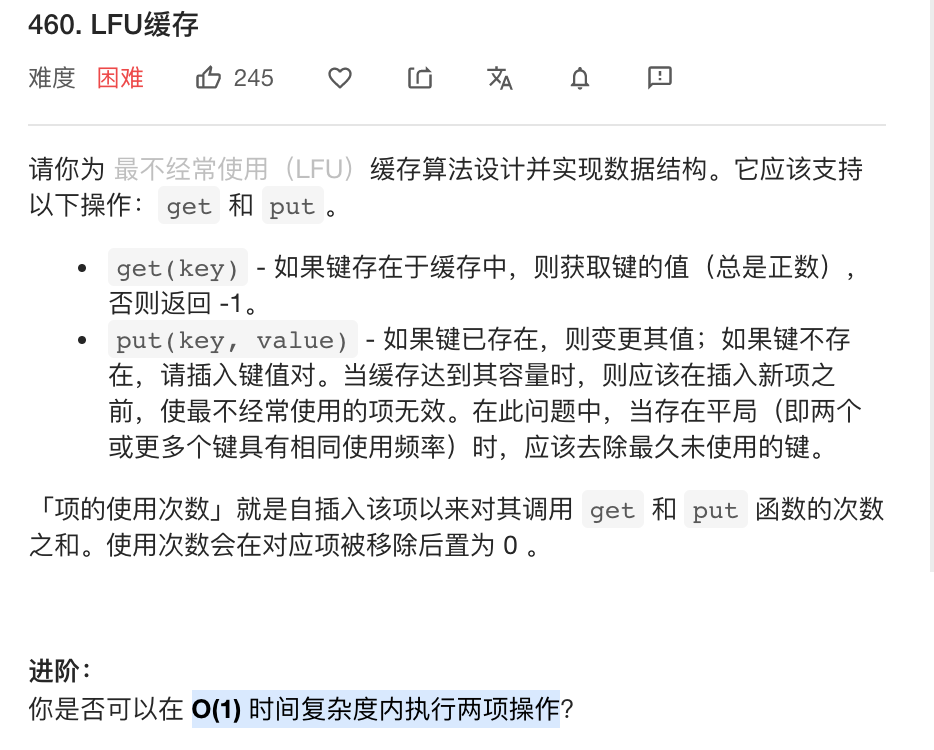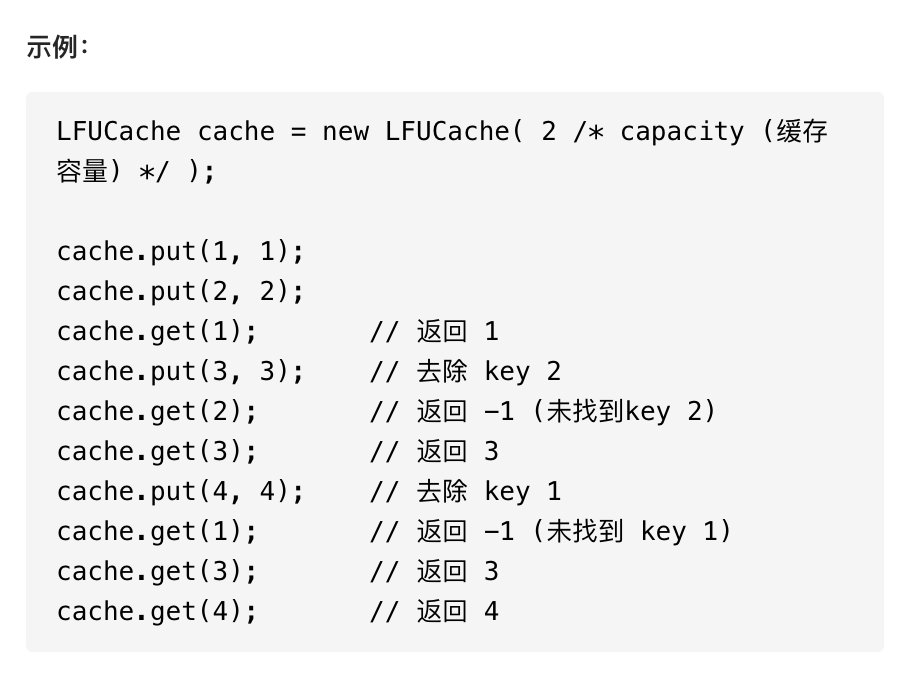## 二、分析

### 方法一：哈希表 + 平衡二叉树

•  因为我们刚刚分析过，LFU算法关系到数据的访问频次和数据的新旧(即数据的访问时间)，所以我们封装一个数据结构：
struct Node
{
//数据的访问频次
int cnt;

//数据的访问时间
int time;

//数据
int key, value;

// 我们需要实现一个 Node 类的比较函数
// 将 cnt（使用频率）作为第一关键字，time（最近一次使用的时间）作为第二关键字
// 因为我们要在数据频率相等时淘汰数据旧的，也就是时间小的
bool operator< (const Node& rhs) const
{
return cnt == rhs.cnt ? time < rhs.time : cnt < rhs.cnt;
}
};

•  比较直观的想法就是我们用哈希表 key_table 以键 key 为索引存储缓存根据 (cnt，time) 双关键字建立一个平衡二叉树 S 来保持缓存
•  由于在 C++ 中，我们可以使用 STL 提供的 std::set 类，set 背后的实现是红黑树：
• 对于 get(key) 操作，我们只要查看一下哈希表 key_table 是否有 key 这个键即可，有的话需要同时更新哈希表和集合中该缓存的使用频率以及使用时间，否则返回 -1。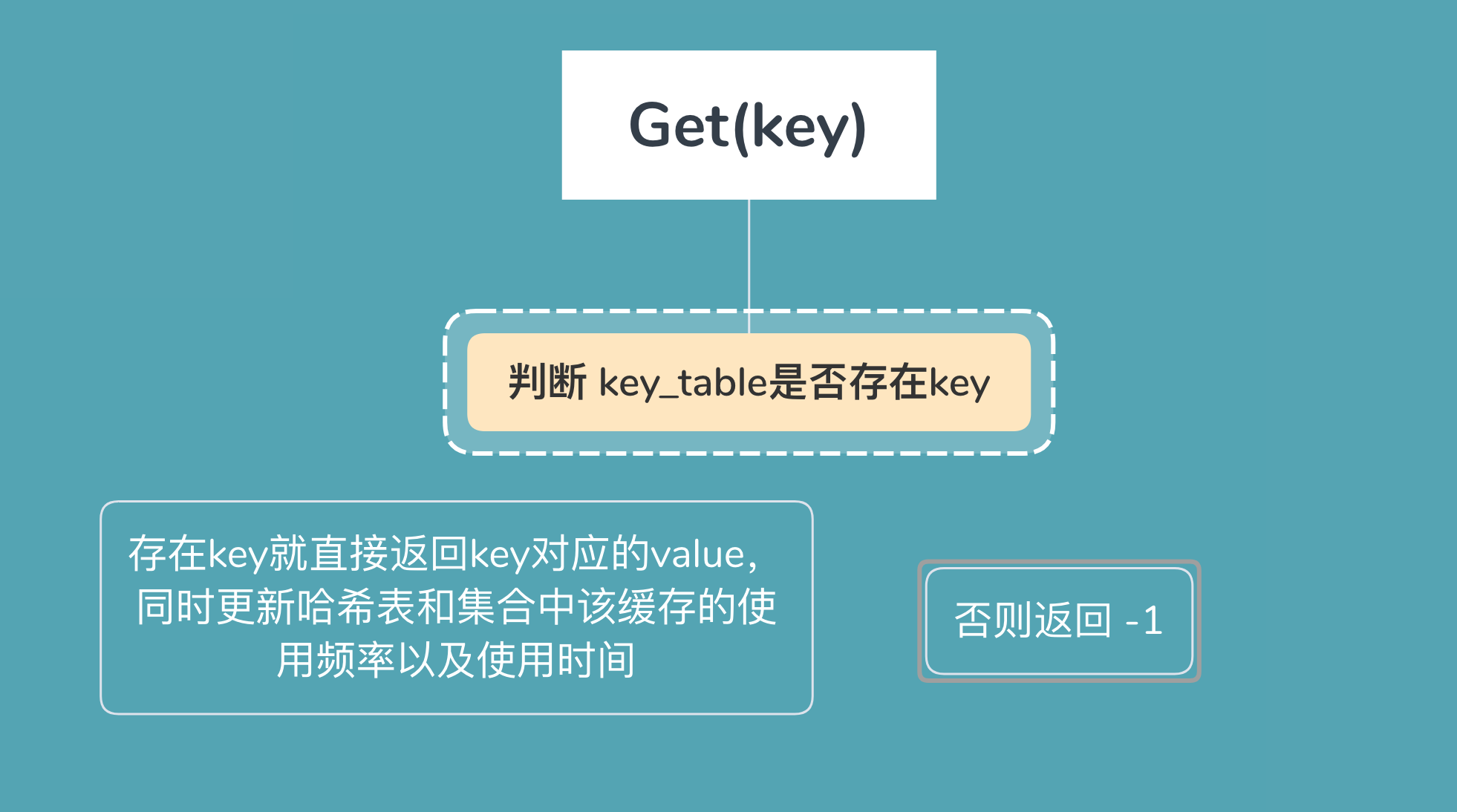• 对于 put(key, value) 操作，首先需要查看 key_table 中是否已有对应的键值如果有的话操作基本等同于 get(key)，不同的是需要更新缓存的 value 值。如果没有的话相当于是新插入一个缓存，这时候需要先查看是否达到缓存容量 capacity，如果达到了的话，需要删除最近最少使用的缓存，即平衡二叉树中最左边的结点，同时删除 key_table 中对应的索引，最后向 key_table 和 S 插入新的缓存信息即可。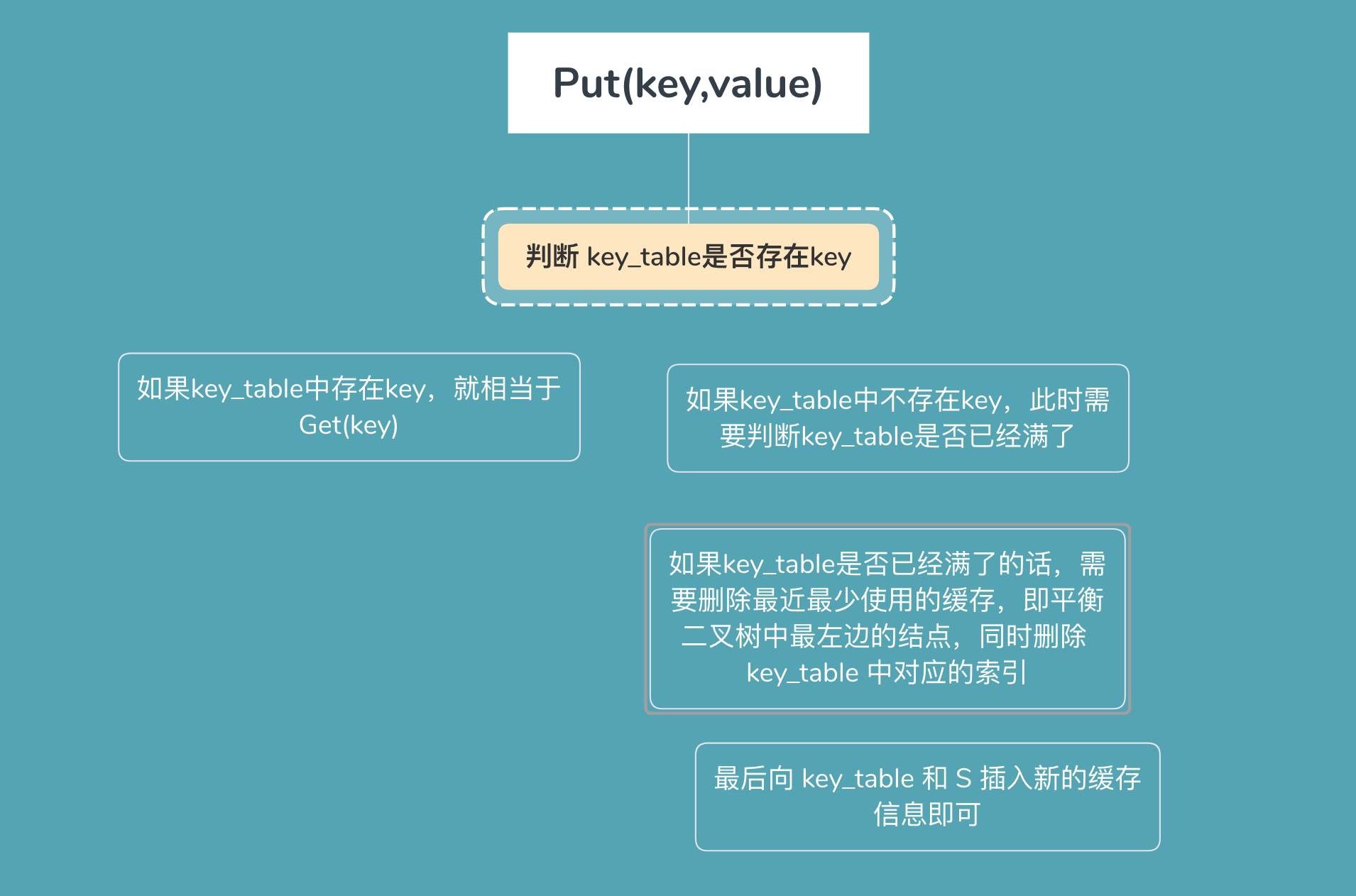•  完整代码
struct Node
{
//频率
int cnt;

//时间
int time;

//数据
int key, value;

//构造
Node(int _cnt, int _time, int _key, int _value)
:cnt(_cnt)
, time(_time)
, key(_key)
, value(_value)
{}

//自定义比较规则：以频率为第一关键字，时间为第二关键字
bool operator < (const Node& rhs) const
{
return cnt == rhs.cnt ? time < rhs.time : cnt < rhs.cnt;
}
};

class LFUCache
{
// 缓存容量
int capacity;

//时间戳
int time;

//关键字和数据信息的哈希索引
unordered_map<int, Node> key_table;

//平衡搜索树
set<Node> S;

public:
LFUCache(int _capacity)
{
capacity = _capacity;
time = 0;
key_table.clear();
S.clear();
}

int get(int key)
{
if (capacity == 0)
return -1;

auto it = key_table.find(key);
// 如果哈希表中没有键 key，返回 -1
if (it == key_table.end())
return -1;

// 从哈希表中得到旧的缓存
Node cache = it -> second;

// 从平衡二叉树中删除旧的缓存
S.erase(cache);

// 将旧缓存更新
cache.cnt += 1;
cache.time = ++time;

// 将新缓存重新放入哈希表和平衡二叉树中
S.insert(cache);

//更新哈希表当中key对应的缓存数据
it -> second = cache;

//返回
return cache.value;
}

void put(int key, int value)
{
if (capacity == 0)
return;

auto it = key_table.find(key);
if (it == key_table.end())
{
// 如果到达缓存容量上限
if (key_table.size() == capacity)
{
// 从哈希表和平衡二叉树中删除最近最少使用的缓存
key_table.erase(S.begin() -> key);
S.erase(S.begin());
}

// 创建新的缓存
Node cache = Node(1, ++time, key, value);

// 将新缓存放入哈希表和平衡二叉树中
key_table.insert(make_pair(key, cache));
S.insert(cache);
}
else
{
// 这里和 get() 函数类似
//代表找到了key，取对应的缓存数据
Node cache = it -> second;

//在红黑树S中删除旧的缓存数据
S.erase(cache);

//在旧的缓存基础上更新缓存数据
cache.cnt += 1;
cache.time = ++time;
cache.value = value;

//重新把缓存数据插到红黑树S当中
S.insert(cache);

//跟新哈希表对应key的缓存数据
it -> second = cache;
}
}
};


### 方法二：双哈希表

•  我们定义两个哈希表

•  第一个 freq_table 以频率 freq 为索引，每个索引存放一个双向链表，这个链表里存放所有使用频率为 freq 的缓存，缓存里存放三个信息，分别为键 key，值 value，以及使用频率 freq。

•  第二个 key_table 以键值 key 为索引，每个索引存放对应缓存在 freq_table 中链表里的内存地址，这样我们就能利用两个哈希表来使得两个操作的时间复杂度均为 O(1)。

•  同时需要记录一个当前缓存最少使用的频率 minFreq，这是为了删除操作服务的。

•  对于 get(key) 操作，我们能通过索引 key 在 key_table 中找到缓存在 freq_table 中的链表的内存地址，如果不存在直接返回 -1，否则我们能获取到对应缓存的相关信息，这样我们就能知道缓存的键值还有使用频率，直接返回 key 对应的值即可。

•  但是我们注意到 get 操作后这个缓存的使用频率加一了，所以我们需要更新缓存在哈希表 freq_table 中的位置。

•  已知这个缓存的键 key，值 value，以及使用频率 freq，那么该缓存应该存放到 freq_table 中 freq + 1 索引下的链表中

•  所以我们在当前链表中 删除该缓存对应的节点，根据情况更新 minFreq 值，然后将其插入到 freq + 1 索引下的链表头完成更新。这其中的操作复杂度均为 O(1)。

•  你可能会疑惑更新的时候为什么是插入到链表头，这其实是为了保证缓存在当前链表中从链表头到链表尾的插入时间是有序的，为下面的删除操作服务。

•  对于 put(key, value) 操作，我们先通过索引 key在 key_table 中查看是否有对应的缓存，如果有的话，其实操作等价于 get(key) 操作，唯一的区别就是我们需要将当前的缓存里的值更新为 value。如果没有的话，相当于是新加入的缓存，如果缓存已经到达容量，需要先删除最近最少使用的缓存，再进行插入

•  先考虑插入，由于是新插入的，所以缓存的使用频率一定是 1，所以我们将缓存的信息插入到 freq_table 中 1 索引下的列表头即可，同时更新 key_table[key] 的信息，以及更新 minFreq = 1。

•  那么剩下的就是删除操作了，由于我们实时维护了 minFreq，所以我们能够知道 freq_table 里目前最少使用频率的索引同时因为我们保证了链表中从链表头到链表尾的插入时间是有序的，所以 freq_table[minFreq] 的链表中链表尾的节点即为使用频率最小且插入时间最早的节点，我们删除它同时根据情况更新 minFreq ，整个时间复杂度均为O(1)。

•  完整代码

// 缓存的节点信息
struct Node
{
//数据
int key, val;

//频率
int freq;

Node(int _key,int _val,int _freq)
: key(_key)
, val(_val)
, freq(_freq)
{}

};
class LFUCache
{
//缓存的最小频率
int minfreq;

//容量
int capacity;

//哈希表；key为关键字key，value为key在freq_table中的位置
unordered_map<int, list<Node>::iterator> key_table;

//哈希表；key为缓存数据的频率，value为缓存数据的链
unordered_map<int, list<Node>> freq_table;

public:
LFUCache(int _capacity)
{
minfreq = 0;
capacity = _capacity;
key_table.clear();
freq_table.clear();
}

int get(int key)
{
if (capacity == 0)
return -1;

auto it = key_table.find(key);
//没找到就直接返回
if (it == key_table.end())
return -1;

//代表找到了，取key对应的缓存数据在freq_table中某条链上的位置
list<Node>::iterator node = it -> second;

//保存旧的缓存数据
int val = node -> val, freq = node -> freq;

//把旧的缓存数据从freq_table对应频率的链上删除
freq_table[freq].erase(node);

// 如果删除后，当前链表为空，我们需要在哈希表中删除，且更新minFreq
if (freq_table[freq].size() == 0)
{
freq_table.erase(freq);
if (minfreq == freq)
minfreq += 1;
}

// 插入到 freq + 1 中频率的链头，因为该缓存数据get了一次
freq_table[freq + 1].push_front(Node(key, val, freq + 1));

//同时更新该缓存数据在key_table中的信息
key_table[key] = freq_table[freq + 1].begin();

return val;
}

void put(int key, int value)
{
if (capacity == 0)
return;
auto it = key_table.find(key);
//如果没找到该缓存数据，就需要插入缓存，但是需要判断满否？
if (it == key_table.end())
{
// 如果缓存已满，需要进行删除操作
if (key_table.size() == capacity)
{
// 通过最小缓存频率 minFreq 拿到其在 freq_table[minFreq] 链表的末尾节点
auto it2 = freq_table[minfreq].back();

//把使用最少最旧的缓存数据在key_table和freq_table中淘汰掉
key_table.erase(it2.key);
freq_table[minfreq].pop_back();

//如果删除后对应频率的链表为空，也需要在freq_table中删除
if (freq_table[minfreq].size() == 0)
{
freq_table.erase(minfreq);
}
}

//执行插入缓存操作：在freq_table中频率为1的链头插入新的缓存数据
freq_table.push_front(Node(key, value, 1));

//在key_table中保存该缓存数据在链的迭代器
key_table[key] = freq_table.begin();

//更新最小的频率
minfreq = 1;
}
else
{
//代表找到了缓存数据
// 与 get 操作基本一致，除了需要更新缓存的值
//取该缓存数据在freq_table中某条链的迭代器
list<Node>::iterator node = it -> second;

//从迭代器中取到该缓存的频率
int freq = node -> freq;

//从freq_table中对应链删除该缓存
freq_table[freq].erase(node);

//同样需要判断删除后该链是否为空
if (freq_table[freq].size() == 0)
{
freq_table.erase(freq);
if (minfreq == freq)
minfreq += 1;
}

//把该缓存数据放大freq_table的频率 + 1 的链头
freq_table[freq + 1].push_front(Node(key, value, freq + 1));

//同时更新该缓存数据在key_table中的位置
key_table[key] = freq_table[freq + 1].begin();
}
}
};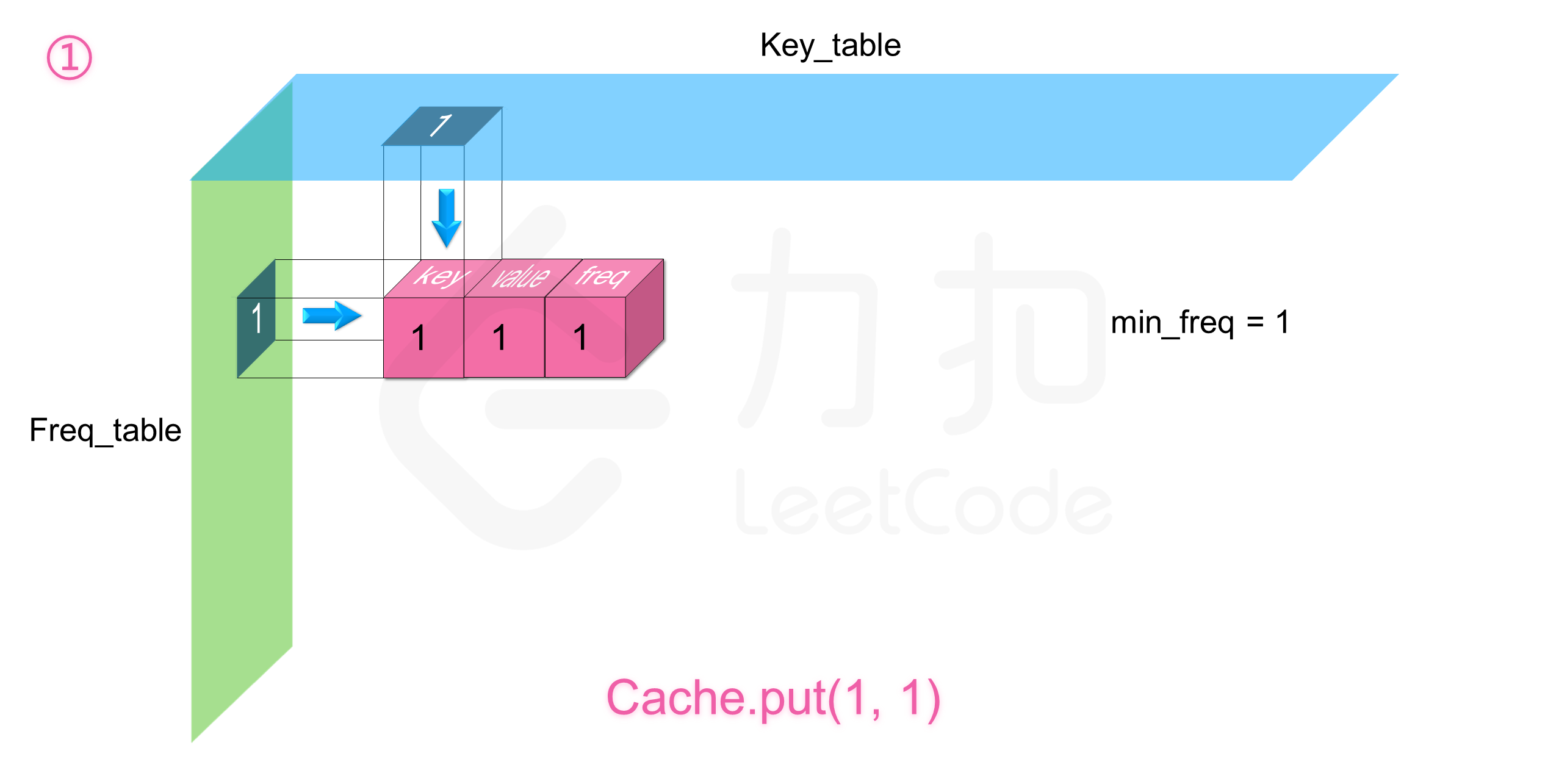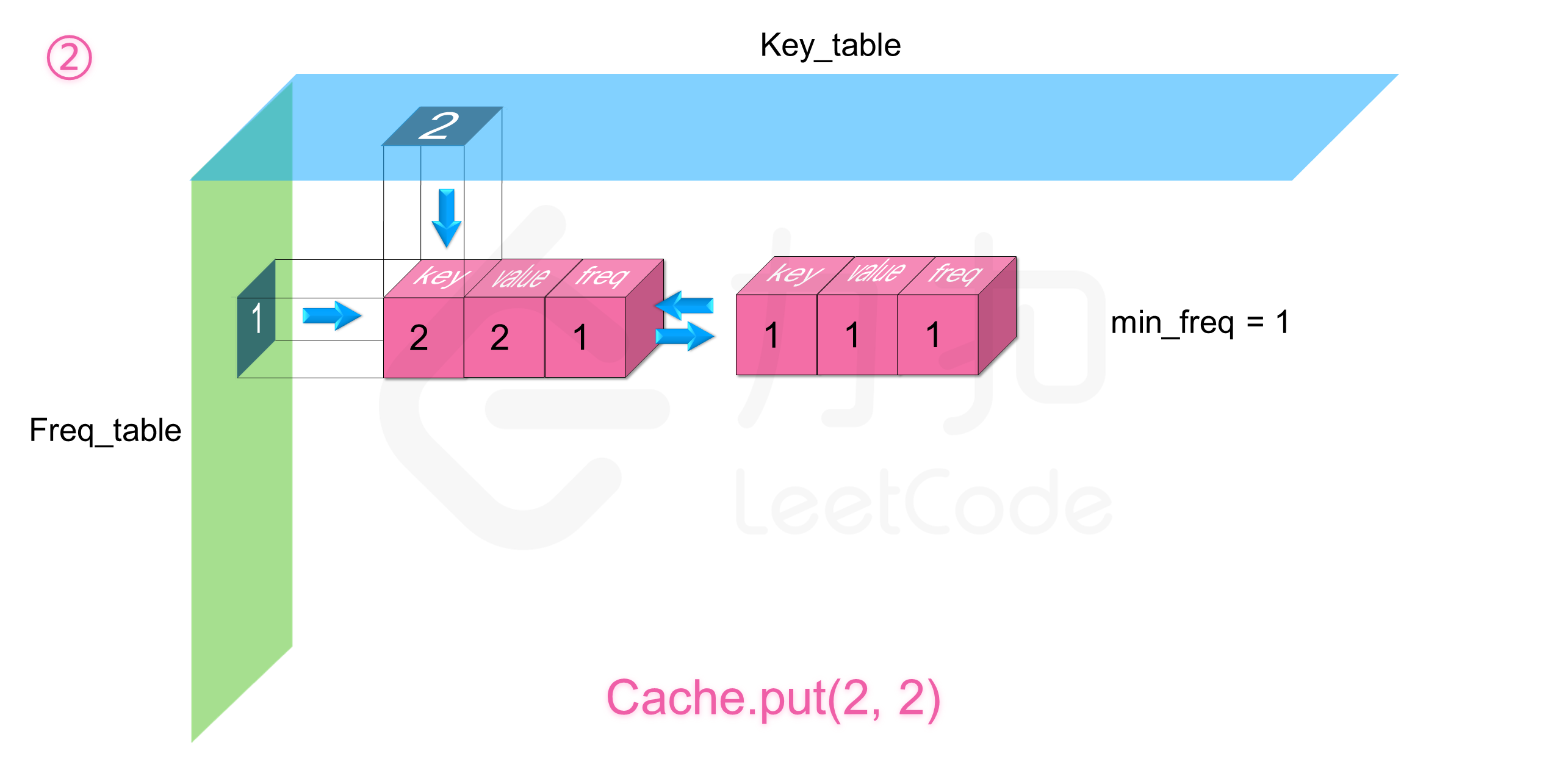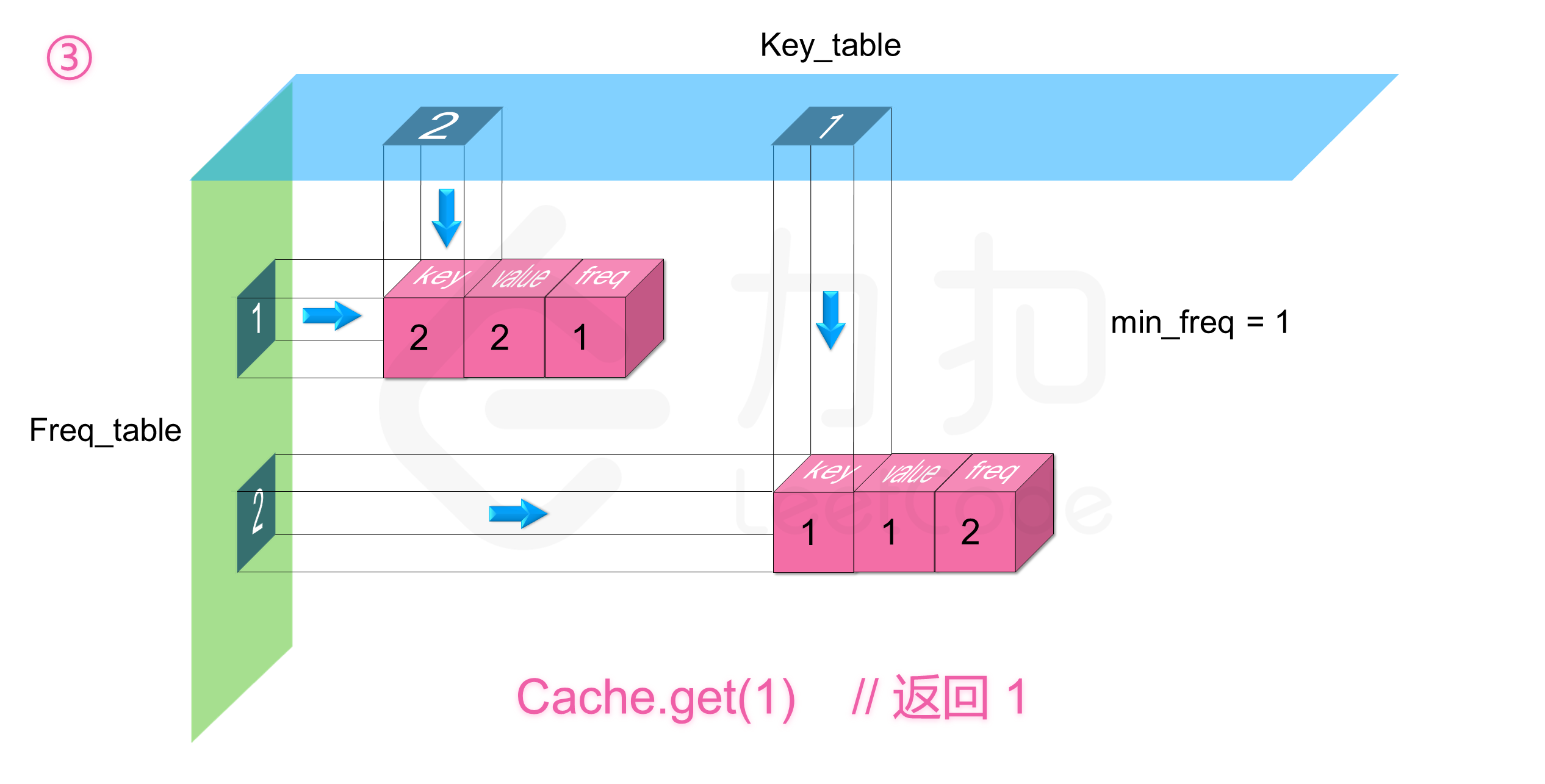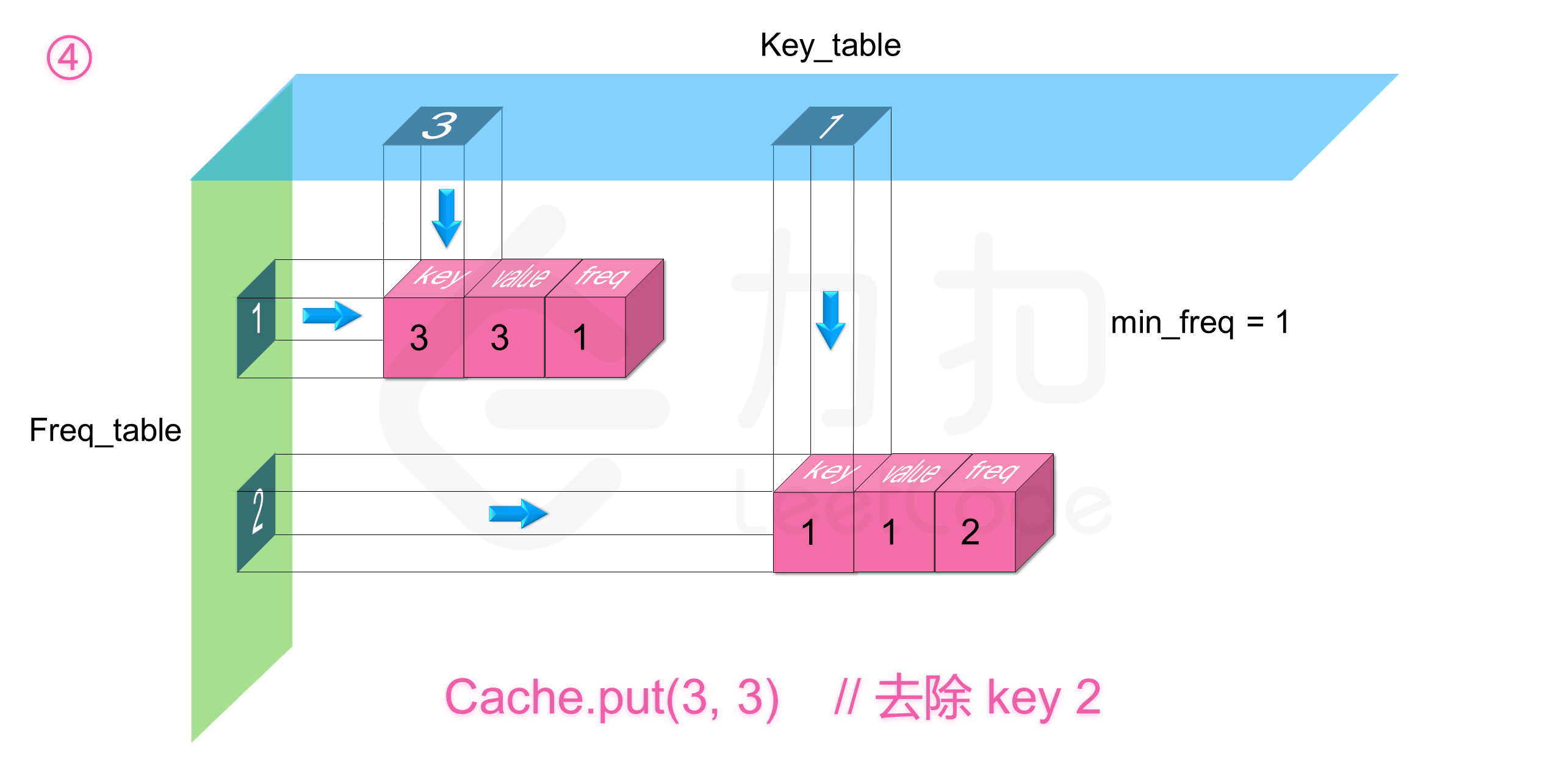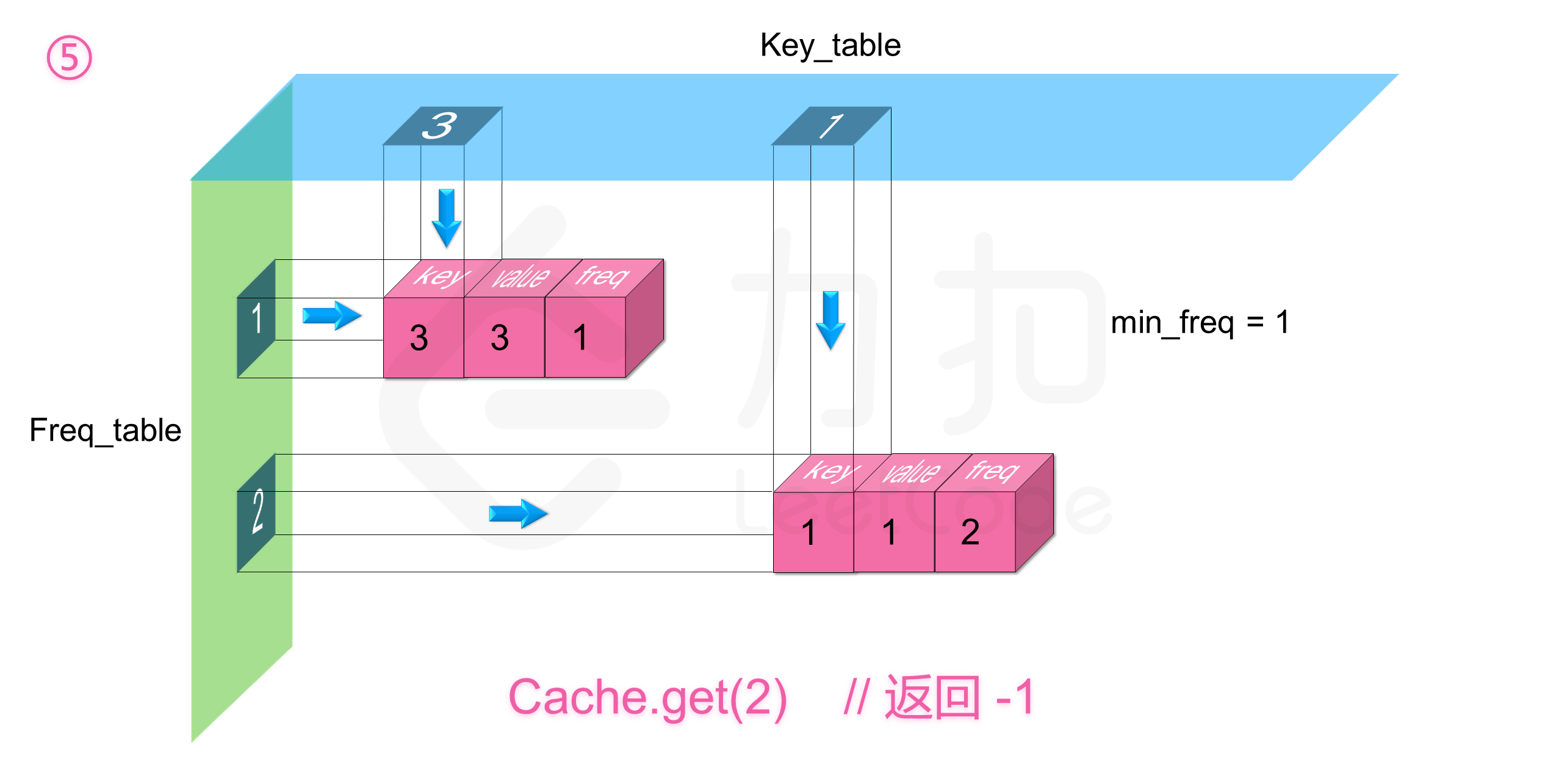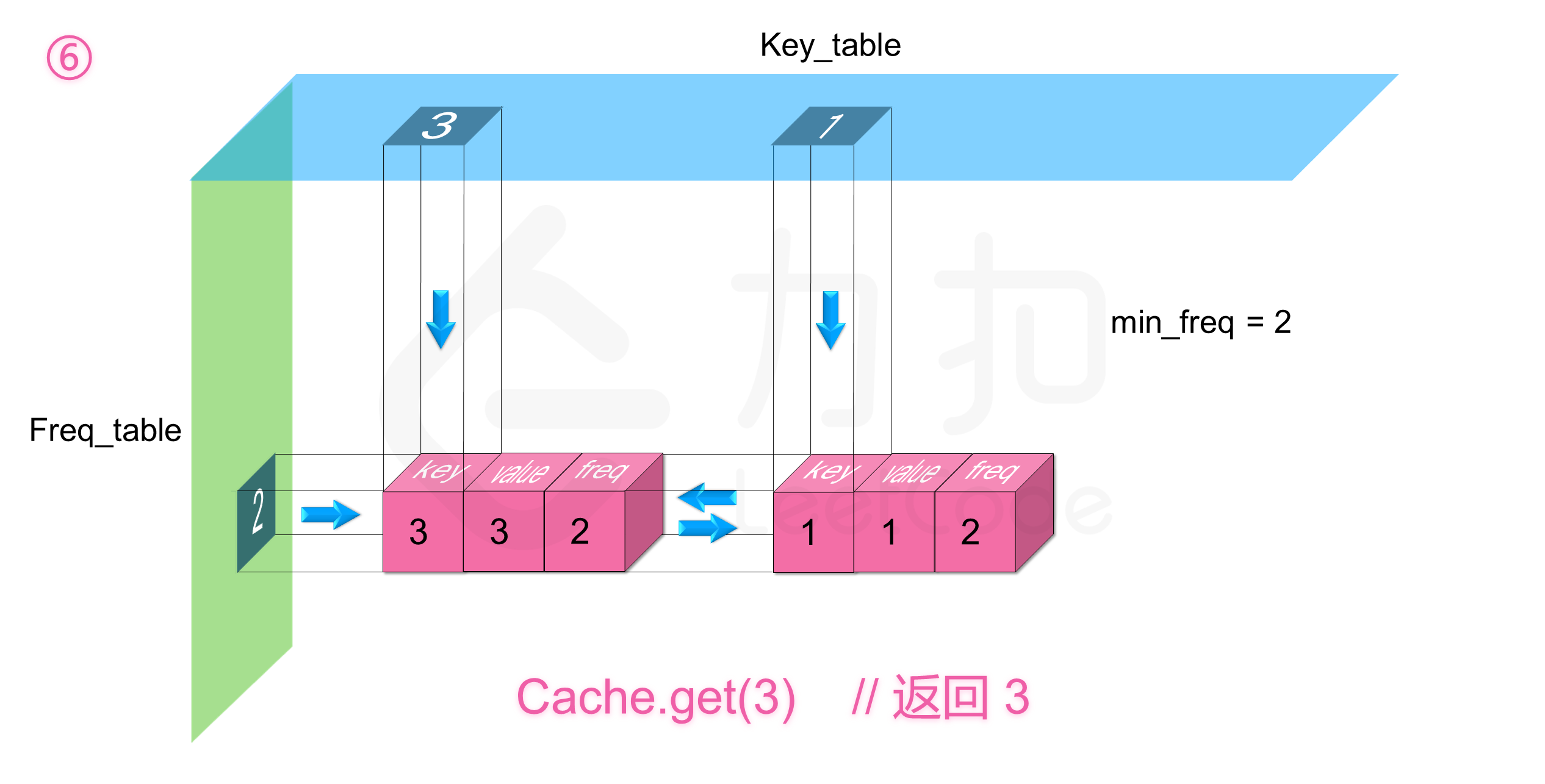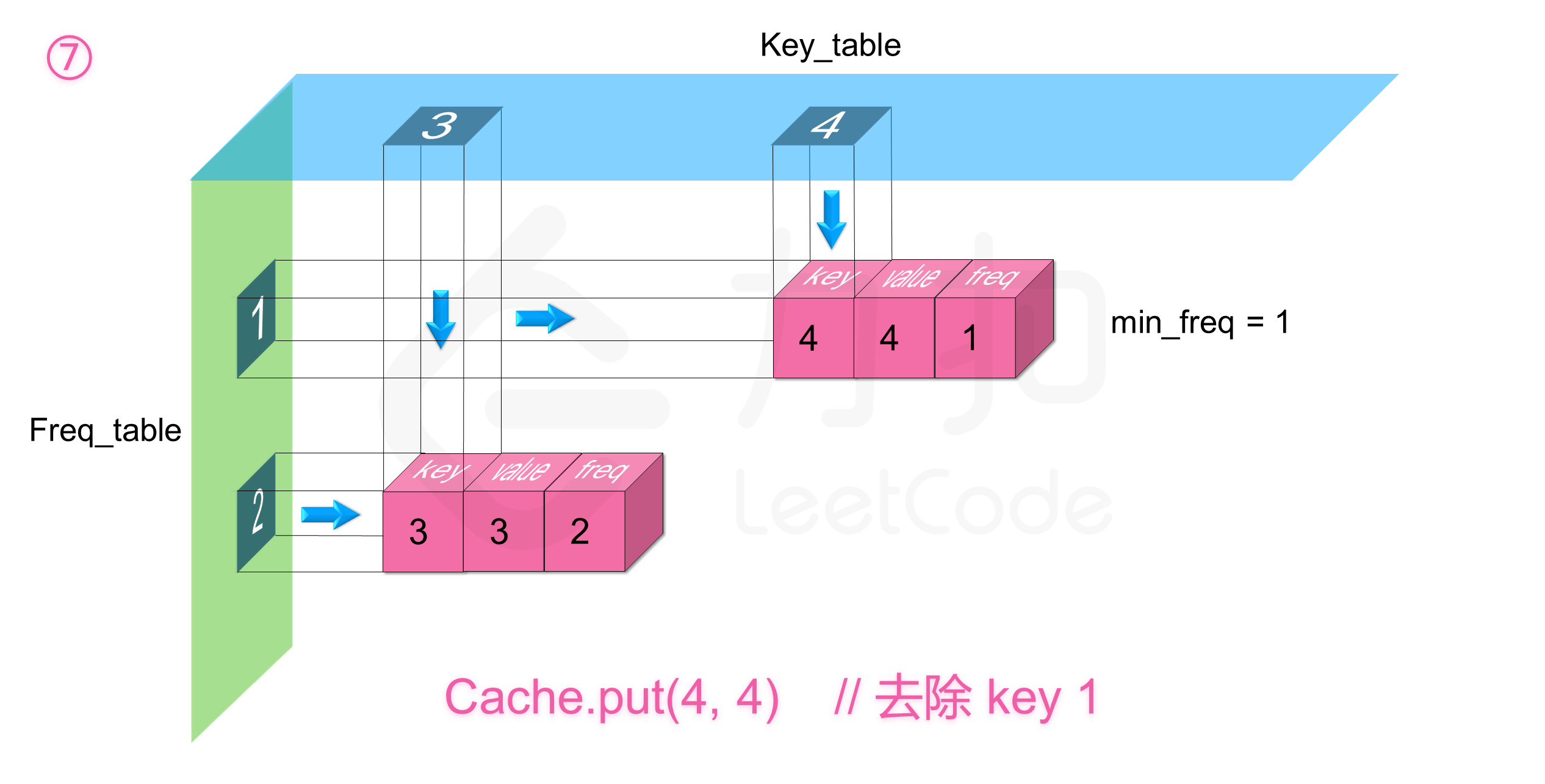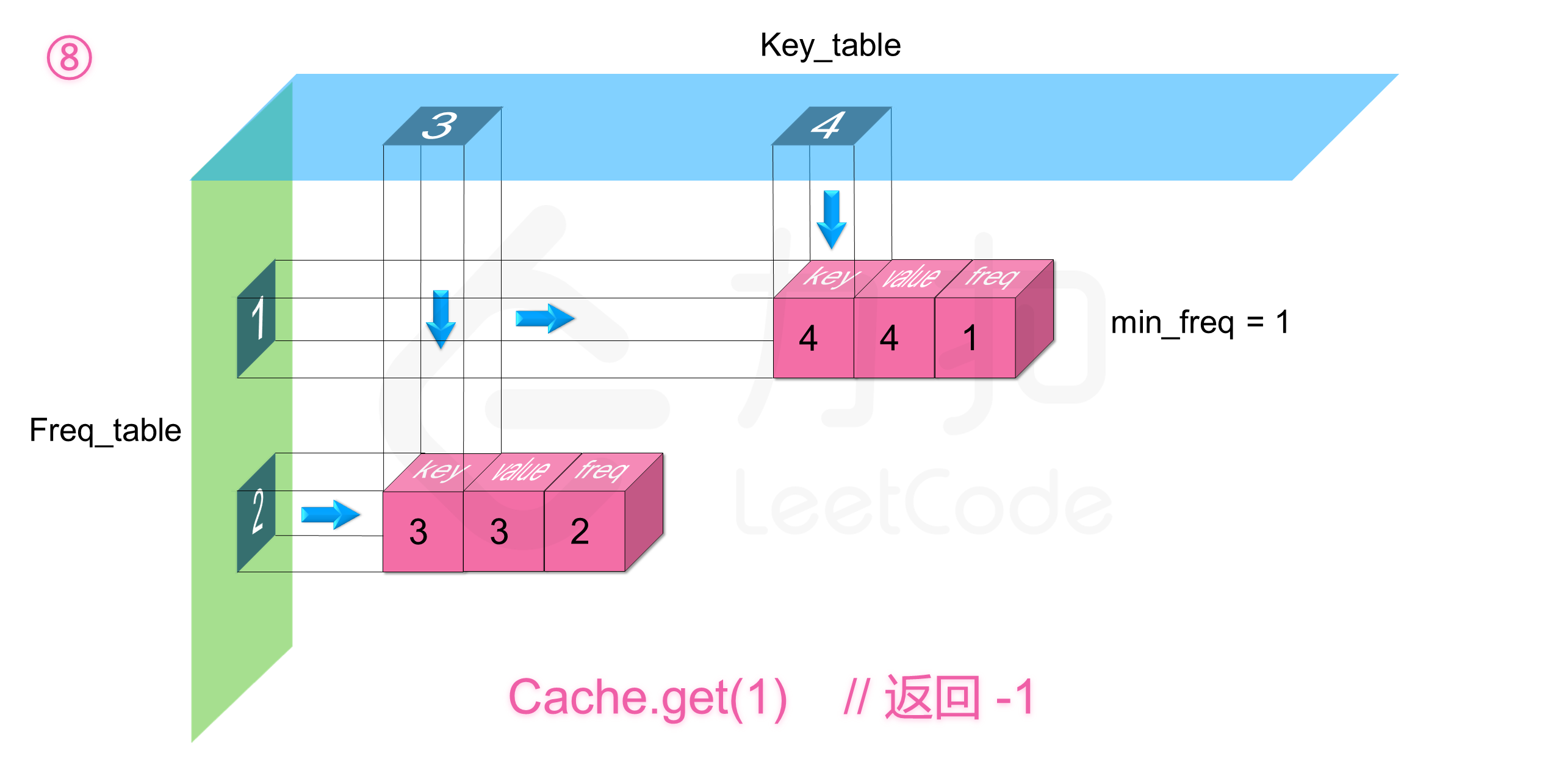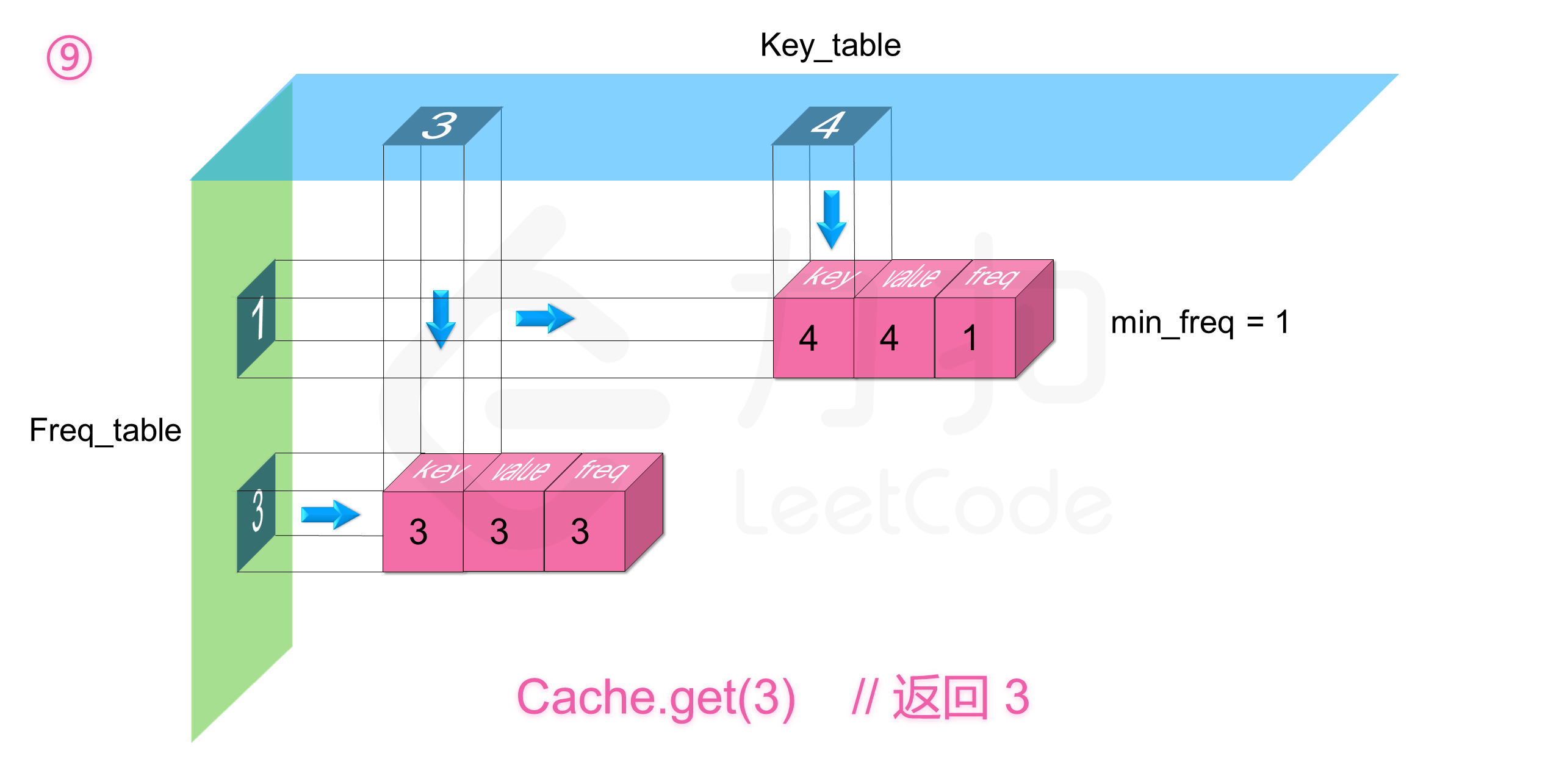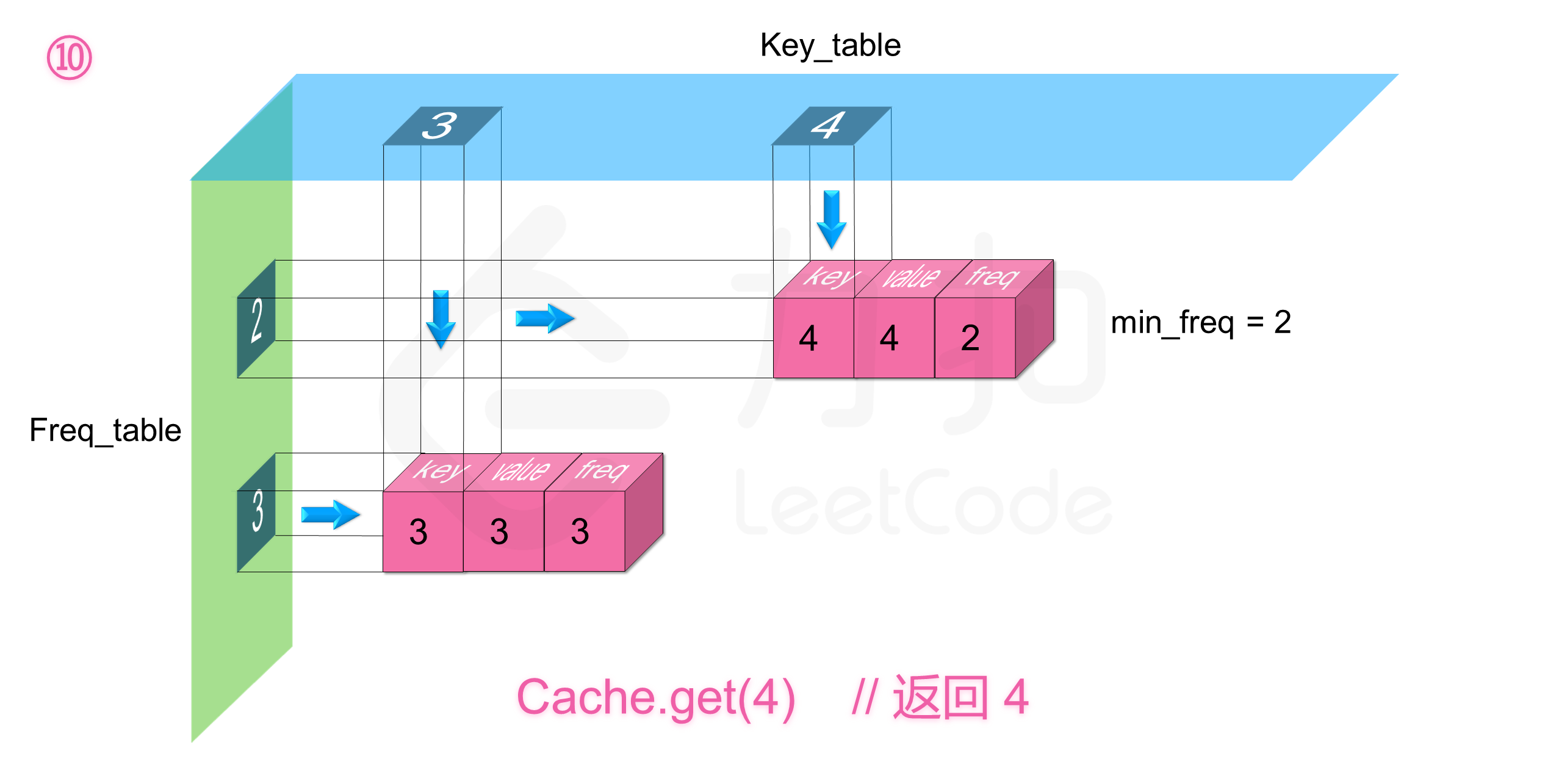0